# Dividing Rational Numbers Lesson Plan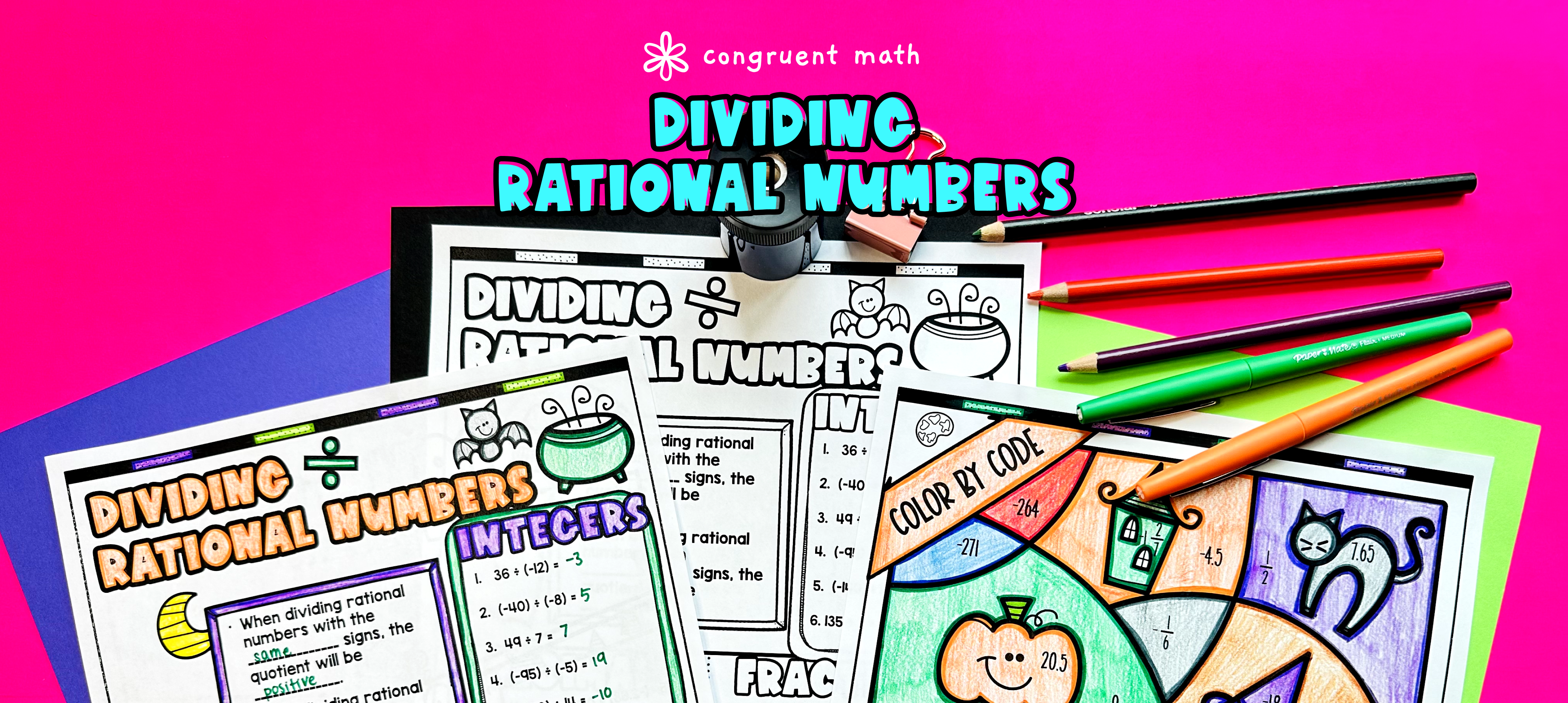Pin This

## Overview

Ever wondered how to teach dividing rational numbers, including fractions, integers, and decimals, in an engaging way to your middle school students?

In this lesson plan, students will learn about dividing rational numbers and their real-life applications. Through artistic and interactive guided notes, check for understanding questions, a color by code activity, and a maze worksheet, students will gain a comprehensive understanding of dividing rational numbers.

The lesson culminates with a real-life example that explores how dividing rational numbers can be applied to splitting a bill at a restaurant.

## Get the Lesson Materials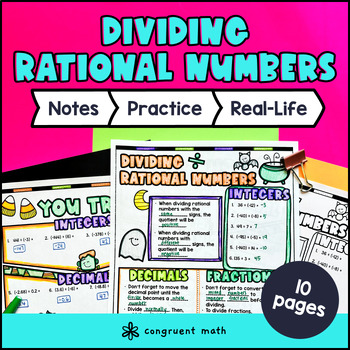\$4.25

## Learning Objectives

After this lesson, students will be able to:

• Divide rational numbers, including fractions, integers, and decimals

• Solve division problems involving positive and negative rational numbers

• Apply division of rational numbers to real-life situations

## Prerequisites

Before this lesson, students should be familiar with:

• Basic operations with rational numbers (adding, subtracting, and multiplying)

• Basic understanding of fractions and decimals

• Knowledge of how to determine the greatest common factor (GCF) and least common multiple (LCM) of numbers

## Key Vocabulary

• Rational numbers

• Fractions

• Decimals

• Integers

• Division

## Procedure

### Introduction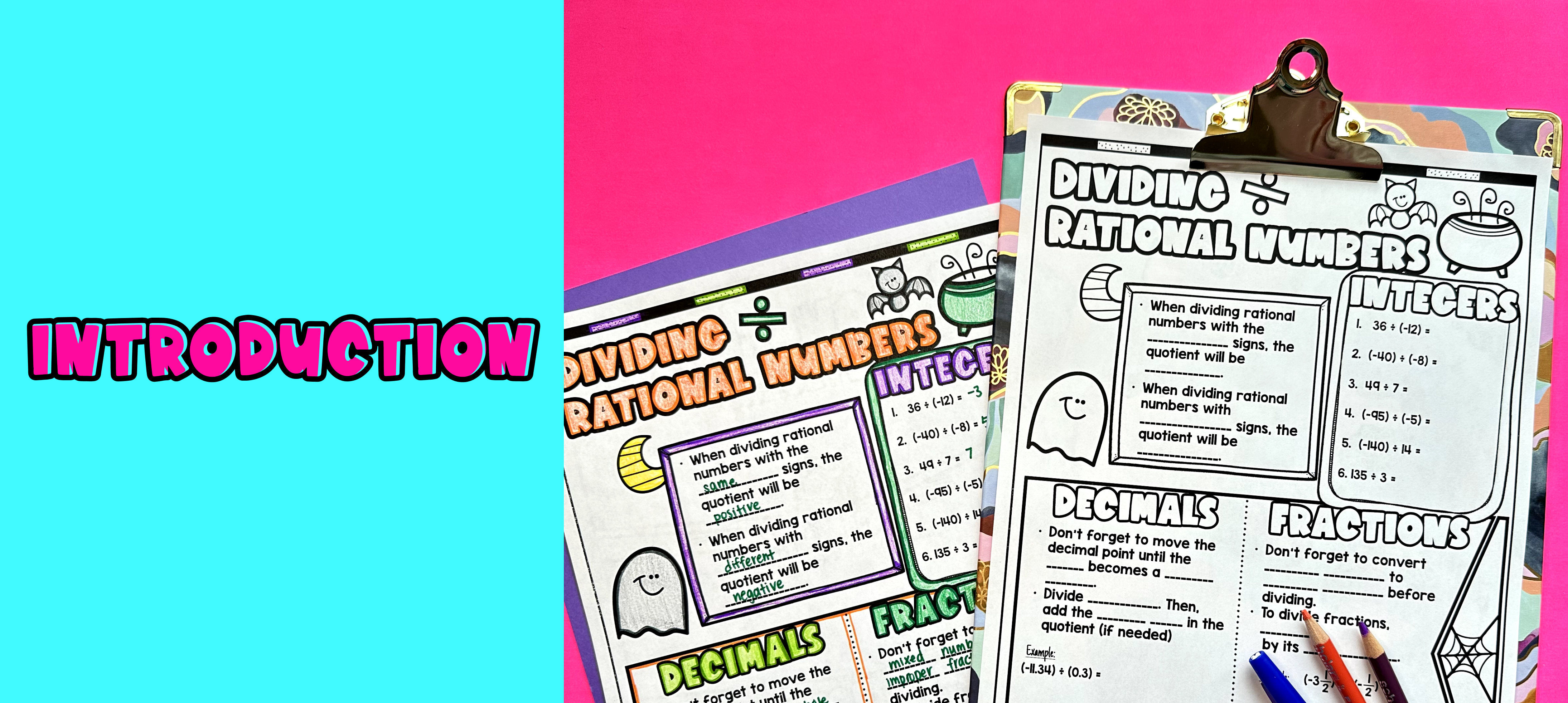Pin This

As a hook, ask students why dividing rational numbers, including fractions, integers, and decimals, is important in real life. Refer to the real-life math application on the last page of the guided notes as well as the FAQs below for ideas.

Use the guided notes to introduce the concept of dividing rational numbers. Walk through the key points of the topic, including the steps and techniques involved in dividing rational numbers. Refer to the FAQ below for a walk through on this, as well as ideas on how to respond to common student questions.

• Check for Understanding: Have students walk through the "You Try!" section of the guided notes. Call on students to talk through their answers, potentially on the whiteboard or projector. Based on student responses, reteach concepts that students need extra help with.

Based on student responses, reteach concepts that students need extra help with. If your class has a wide range of proficiency levels, you can pull out students for reteaching, and have more advanced students begin work on the practice exercises.

### Practice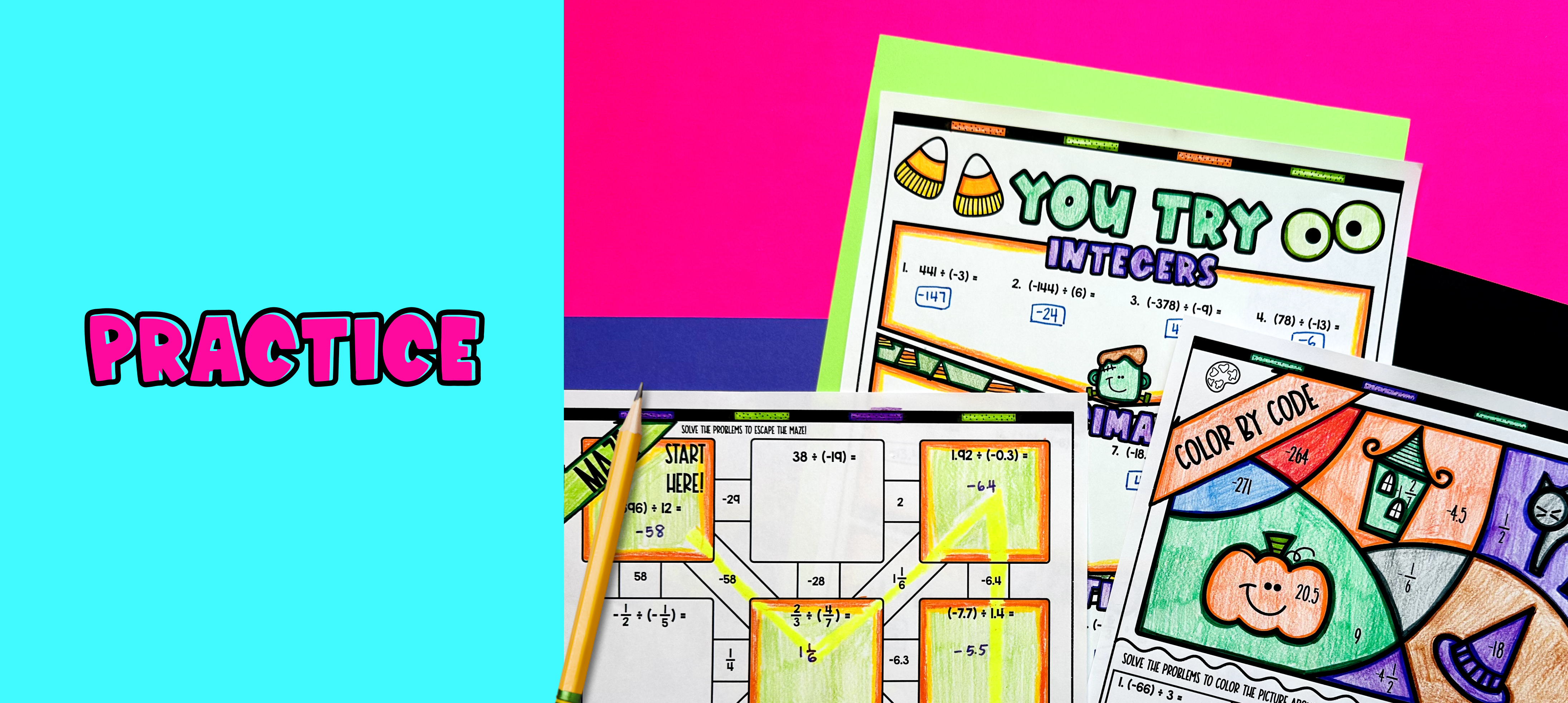Pin This

Have students practice dividing rational numbers including fractions, integers, and decimals using the color by code activity included in the resource. Walk around the classroom to answer any student questions and provide assistance as needed.

Fast finishers can work on the maze activity for extra practice. You can assign these activities as homework for the remainder of the class.

### Real-Life Application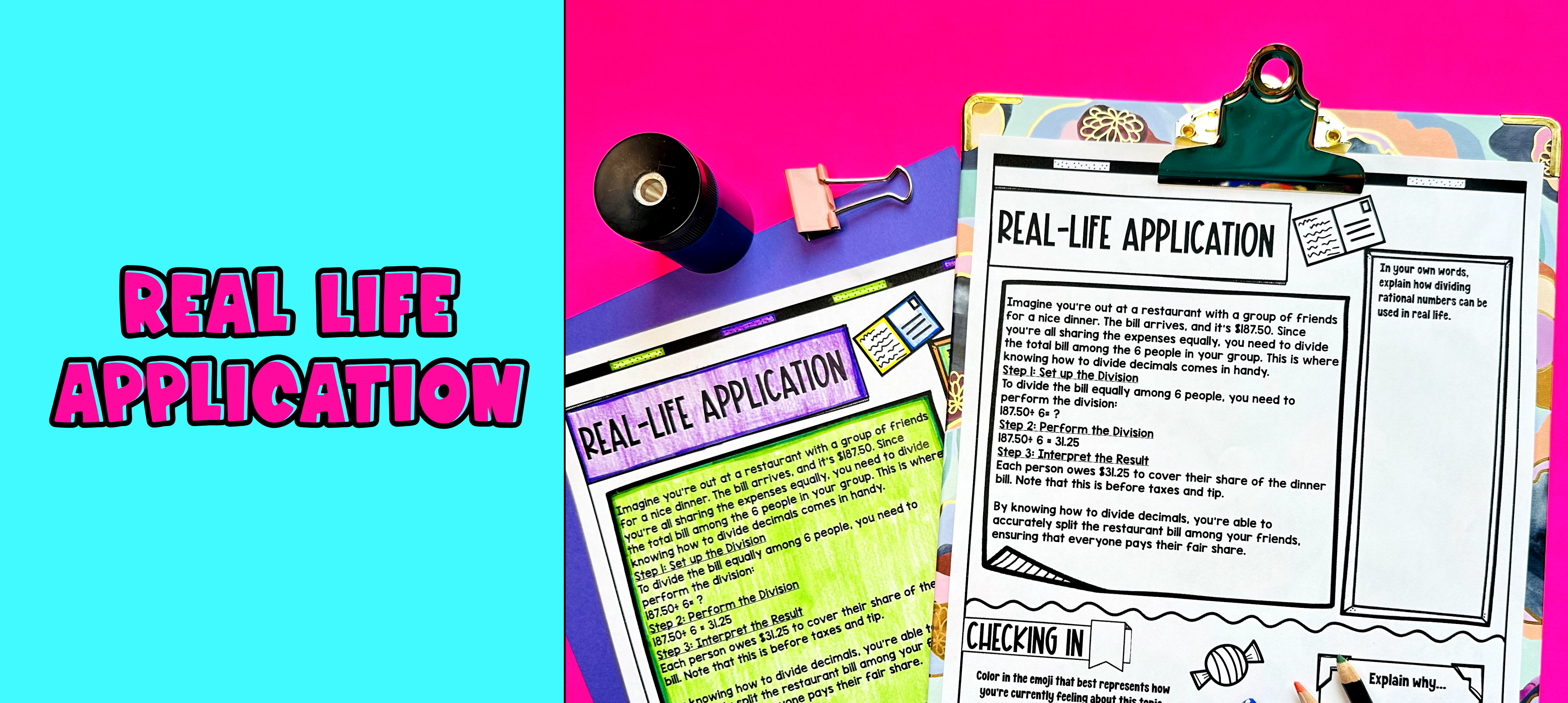Pin This

Bring the class back together, and introduce the concept of rational number division applied to splitting a bill with friends. Refer to the FAQ for more real life applications that you can use for the discussion!

## Extensions

If you’re looking for digital practice for dividing rational numbers, try my Pixel Art activities in Google Sheets. Every answer is automatically checked, and correct answers unlock parts of a mystery picture. It’s incredibly fun, and a powerful tool for differentiation.

Here's an activity to try: